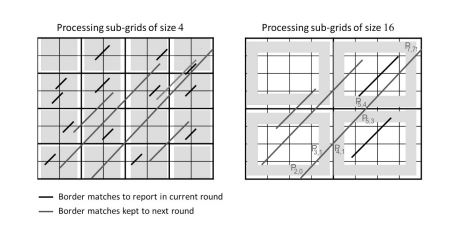Conference Paper

# Practical distributed computation of maximal exact matches in the cloud

By
El-Din S.S.
Aboelhoda M.

Computation of maximal exact matches (MEMs) is an important problem in comparing genomic sequences. Optimal sequential algorithms for computing MEMs have been already introduced and integrated in a number of software tools. To cope with large data and exploit new computing paradigms like cloud computing, it is important to develop efficient and ready-to-use solutions running on distributed parallel architecture. In a previous work, we have introduced a distributed algorithm running on a computer cluster for computing the MEMs. In this paper, we extend this work in two directions: First, we introduce new variants of this algorithm; one of them has a better time complexity than the published one. These variants as we will demonstrate by experiments are faster in practice. Second, we introduce a cloud based implementation, where we automate the process of creating and configuring the cluster, submitting the jobs, and finally collecting the results and terminating the cloud machines. © 2014 IEEE.